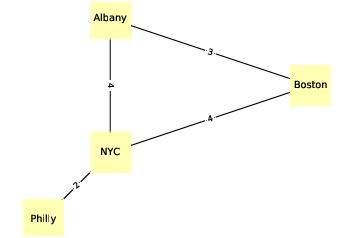# 4.3. NetworkX¶Figure 4.2: An undirected graph that represents driving time between cities.

To represent graphs, we’ll use a package called NetworkX, which is the most commonly used network library in Python.

We can create a directed graph by importing NetworkX (usually imported as `nx`) and instantiating `nx.DiGraph`:

```import networkx as nx
G = nx.DiGraph()
```

At this point, `G` is a `DiGraph` object that contains no nodes and no edges. We can add nodes using the `add_node` method:

```G.add_node('Alice')
```

Now we can use the `nodes` method to get a list of nodes:

```(G.nodes())
NodeView(('Alice', 'Bob', 'Chuck'))
```

The `nodes` method returns a `NodeView`, which can be used in a for loop or, as in this example, used to make a list.

Adding edges works pretty much the same way:

```G.add_edge('Alice', 'Bob')
```

And we can use `edges` to get the list of edges:

```(G.edges())
[('Alice', 'Bob'), ('Alice', 'Chuck'),
('Bob', 'Alice'), ('Bob', 'Chuck')]
```

NetworkX provides several functions for drawing graphs; `draw_circular` arranges the nodes in a circle and connects them with edges:

```(nx.draw_circular(G,
node_color=COLORS,
node_size=2000,
with_labels=True)
```

That’s the code I use to generate Figure 4.1. The option `with_labels` causes the nodes to be labeled; in the next example we’ll see how to label the edges.

To generate Figure 4.2, I start with a dictionary that maps from each city name to its approximate longitude and latitude:

```positions = dict(Albany=(-74, 43),
Boston=(-71, 42),
NYC=(-74, 41),
Philly=(-75, 40))
```

Since this is an undirected graph, I instantiate `nx.Graph`:

```G = nx.Graph()
```

Then I can use `add_nodes_from` to iterate the keys of `positions` and add them as nodes:

```G.add_nodes_from(positions)
```

Next we will make a dictionary that maps from each edge to the corresponding driving time:

```drive_times = {('Albany', 'Boston'): 3,
('Albany', 'NYC'): 4,
('Boston', 'NYC'): 4,
('NYC', 'Philly'): 2}
```

Now I can use `add_edges_from`, which iterates the keys of `drive_times` and adds them as edges:

```G.add_edges_from(drive_times)
```

Instead of `draw_circular`, which arranges the nodes in a circle, we will use `draw`, which takes the position dictionary as the second parameter:

```nx.draw(G, positions,
node_color=COLORS,
node_shape='s',
node_size=2500,
with_labels=True)
```

`draw` uses `positions` to determine the locations of the nodes.

To add the edge labels, we use `draw_networkx_edge_labels`:

```x.draw_networkx_edge_labels(G, positions,
edge_labels=drive_times)
```

The `edge_labels` parameter expects a dictionary that maps from each pair of nodes to a label; in this case, the labels are driving times between cities. And that’s how I generated Figure 4.2.

In both of these examples, the nodes are strings, but in general they can be any hashable type.